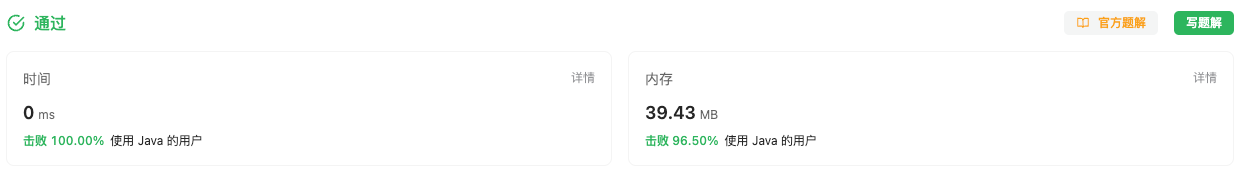#### 2562. 找出数组的串联值

###### 来源: 每日一题 2023.10.12

`nums`串联值 最初等于 `0` 。执行下述操作直到 `nums` 变为空：

``````输入：nums = [7,52,2,4]

- 在第一步操作中：

- 在第二步操作中：

``````

``````输入：nums = [5,14,13,8,12]

- 在第一步操作中：

- 在第二步操作中：

- 在第三步操作中：
nums 只有一个元素，所以我们选中 13 并将其加到串联值上，所以串联值等于 673 。

``````

• `1 <= nums.length <= 1000`
• `1 <= nums[i] <= 10^4`
``````class Solution {
public long findTheArrayConcVal(int[] nums) {

}
}
``````

#### 分析与题解

• 双指针移动 + 模拟法

定义一个双指针, 然后从数组的左右两边往中间移动, 一共会有三种情况.

• `left < right` 时, 进行串联操作.

``````String numString = "" + nums[left] + nums[right];
result += Integer.valueOf(numString);
``````
• `left = right` 时, 直接添加当前的元素.

``````result += nums[left];
``````
• `left > right` 时, 停止遍历操作.

``````while(left <= right) {
...
}
``````

接下来, 我们一起看一下整体的实现逻辑, 具体代码如下所示.

``````class Solution {
public long findTheArrayConcVal(int[] nums) {
int left = 0, right = nums.length - 1;
long result = 0;
while(left <= right) {
if(left == right) {
result += nums[left];
} else {
String numString = "" + nums[left] + nums[right];
result += Integer.valueOf(numString);
}
left++;
right--;
}
return result;
}
}
``````

复杂度分析:

• 时间复杂度: O(nlogU), 一次遍历循环, 时间复杂度与数组长度成线性相关. U表示每一个数字的位数, 转换成字符串的时间复杂度为`logU`
• 空间复杂度: O(logU), 数字转字符串的时候需要的空间复杂度为logU.

结果如下所示.• 双指针移动 + 模拟法 (优化)

由于数字转字符串的时间消耗过长, 所以这里写一个函数, 计算 `a` , `b` 两者的 串联值 . 方法也很简单, 这里就不过多叙述了.

``````public long twoNumsSumResult(int a, int b) {
int times = 0;
int end = b;
while (end > 0) {
end /= 10;
times++;
}
return a * (long)Math.pow(10, times) + b;
}
``````

接下来, 我们一起看一下整体的实现逻辑, 具体代码如下所示.

``````class Solution {
public long findTheArrayConcVal(int[] nums) {
int left = 0, right = nums.length - 1;
long result = 0;
while(left <= right) {
if(left == right) {
result += nums[left];
} else {
result += twoNumsSumResult(nums[left], nums[right]);
}
left++;
right--;
}
return result;
}
public long twoNumsSumResult(int a, int b) {
int times = 0;
int end = b;
while (end > 0) {
end /= 10;
times++;
}
return a * (long)Math.pow(10, times) + b;
}
}
``````

复杂度分析:

• 时间复杂度: O(nU), 一次遍历循环, 时间复杂度与数组长度成线性相关. U表示每一个数字的位数, 对于 `b` 来说, 需要遍历来确认 位数 .
• 空间复杂度: O(n), 确认 `b` 的位数时, 需要创建临时变量.

结果如下所示.IT界无底坑洞栋主 欢迎加Q骚扰:676758285